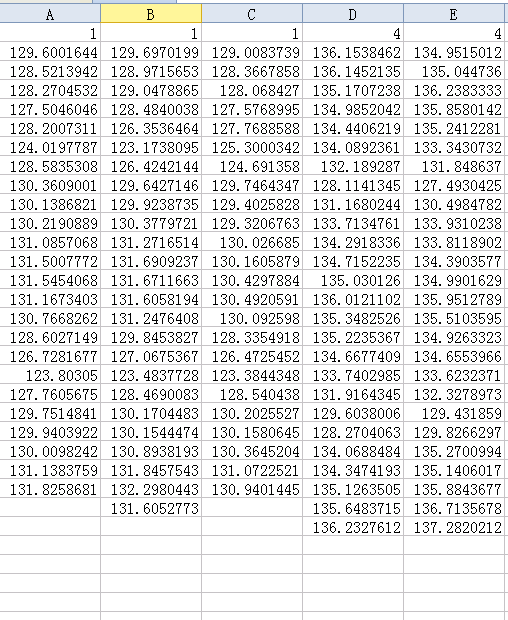num返回filename中的数据部分数组，txt部分用NaN表示

txt返回filename的txt值

raw返回和原数组相同大小的元胞数组

Example：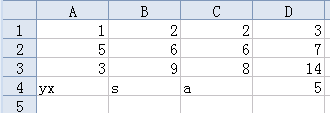>> example

num =

1     2     2     3
5     6     6     7
3     9     8    14
NaN   NaN   NaN     5

txt =

'yx'    's'    'a'

raw =

[ 1]            [ 3]
[ 5]            [ 7]
[ 3]            
'yx'    's'    'a'    [ 5]

Example：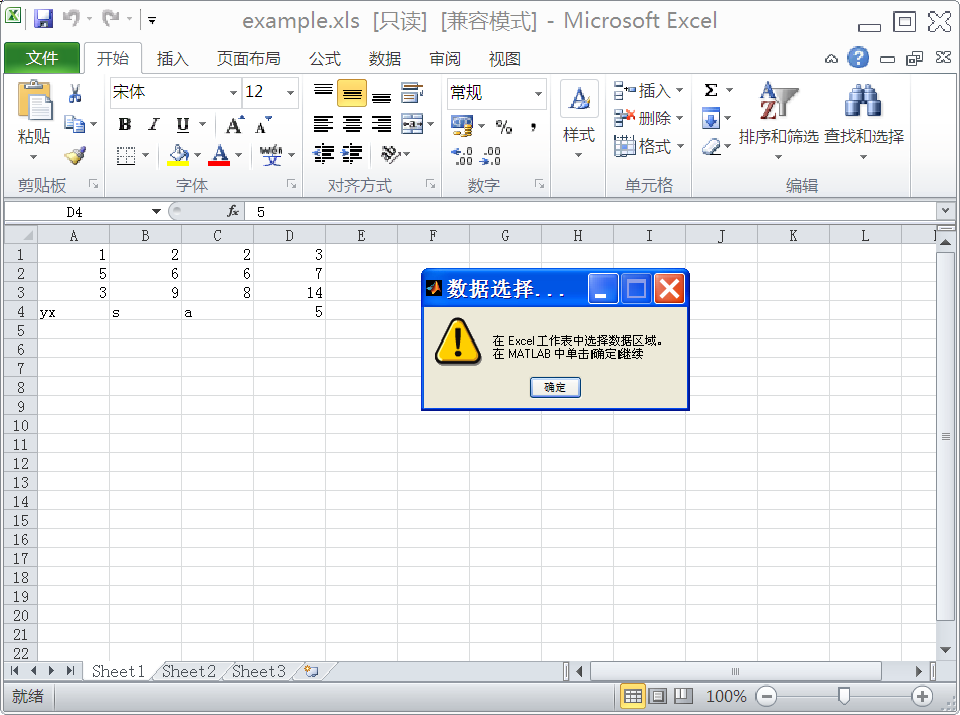Example：

1. 在工作表中执行一个函数 然后返回数值型数据
•       在命令行中输入以下命令 产生一个随机的正态分布矩阵（gallery函数用法参照help）

misc = pi*gallery('normaldata',[10,3],1);
xlswrite('myExample.xlsx',misc,'MyData');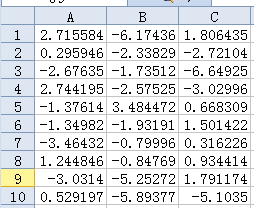• 编写特定功能的函数（此处是将[-3 3]以外的数据变成-3或3，按照最近原则）

function [Data] = setMinMax(Data)

minval = -3; maxval = 3;

for k = 1:Data.Count
v = Data.Value{k};
if v > maxval || v < minval
if v > maxval
Data.Value{k} = maxval;
else
Data.Value{k} = minval;
end
end
end

• 最后按照函数实现对数据的处理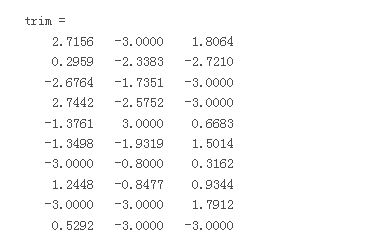（用空字符代替XRange和basic参数，此处用了两个空字符当占位符 placeholders）

2 返回数据的索引（按列计算）

• 函数要满足返回索引的需求

function [Data,indices] = setMinMax(Data)

minval = -3; maxval = 3;
indices = [];

for k = 1:Data.Count
v = Data.Value{k};
if v > maxval || v < minval
if v > maxval
Data.Value{k} = maxval;
else
Data.Value{k} = minval;
end
indices = [indices k];
end
end

• 调用指定函数

>>disp(idx)

>>7     9    11    15    19    20    23    24    30

1  Limitationsxlsread reads only 7-bit ASCII characters（只能读取七位ASCII序列）
2  .xlsread does not support non-contiguous ranges（不支持非邻行的读取，也就是说只能读取相邻的行和列了~~~~(>_<)~~~~ ）

.（选取特定列的方法，待定更新）